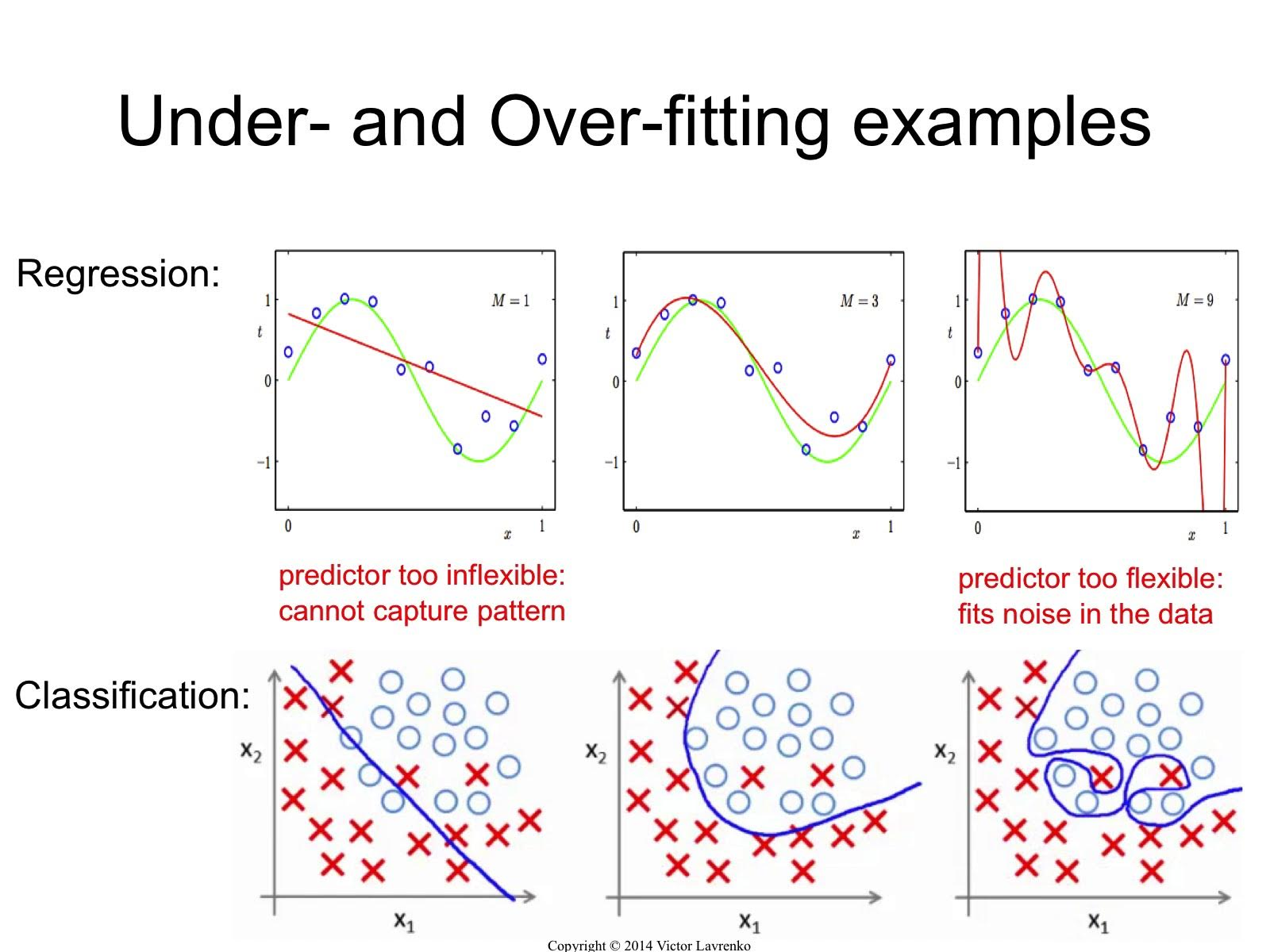Categories:

Updated:

# Underfitting and Overfitting

When the form of our hypothesis function h maps poorly to the trend of the data, we say that our hypothesis is underfitting or has high bias. It is usually caused by a function that is too simple or uses too few features.

Overfitting or high variance is caused by a hypothesis function that fits the available data but does not generalize well to predict new data. It is usually caused by a complicated function that creates a lot of unnecessary curves and angles unrelated to the data.# Why Regularization?

We can prevent overfitting by reducing the number of features or implementing ‘regularization’.

Regularization keeps all the features, but makes parameters $\theta_j$ smaller. It reduces the impact of each features to the predicted output, thus smoothes the regression curve or decision boundary.

Regularization works well when we have a lot of slightly useful features.

# Regularized Linear Regression

## Cost Function

We will penalize increase of theta by adding ‘regularization term’ to cost function.

If theta increases, regularization term $\lambda\Theta^T\Theta$ increases, so cost J increases. We want to minimize cost, so theta has to be smaller than non-regularized regression.

$\lambda$ is the regularization parameter. It determines how much the costs of our theta parameters are inflated.